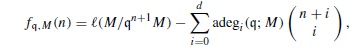# Acta Mathematica Vietnamica

### OPEN ACCESS ISSUES# On Adjusted Hilbert-Samuel Functions Nguyen Tuan Long

## Abstract

Let (R,m ) be a Noetherian local ring and M a finitely generated R-module of dimension d. Let q be a parameter ideal of M. Consider an adjusted Hilbert-Samuel function in n defined bywhere adegi (q ;M)    is the ith arithmetic degree of M with respect to q . In this paper, we prove that if q is a distinguished parameter ideal then there exists an integer n0 such that fq ,M (n)0   for all nn0. Moreover, if M is sequentially generalized Cohen-Macaulay, then n0 exists independently of the choice of q .

You are here: